## Classification

#### Classification

1. Find the odd figure.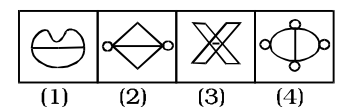1. In all other figures there are two similar designs joined together.

##### Correct Option: A

As we can see that In all other figures there are two similar designs joined together.But , figure ( 1 ) is different to others .

1. In the following question select the one which is different from the other three responses.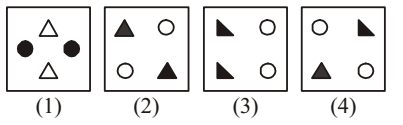1. Except one figure , in all other figures only two types of designs are given.

##### Correct Option: D

Except in figure (4), in all other figures only two types of designs are given.

1. Find the odd figure from the given alternatives.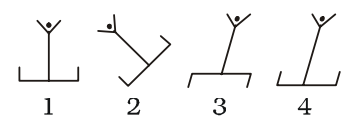1. As we can see that figure (3) is different from the other three.

##### Correct Option: C

As we can see that figure (3) is different from the other three.
The lower line segment is inverted in figure (3).

1. Which figure is different from the rest?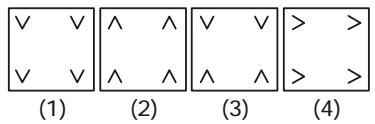1. Except one figure, in all other figures all the four designs face the same direction.

##### Correct Option: C

As we can see that,
Except in figure (3), in all other figures all the four designs face the same direction.

1. In each of the following questions find the odd word/ number/number pair/figure from the given alternatives.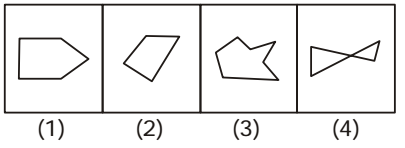1. Except one figure , all others are irregular.
Figure (1) is a regular pentagon.

##### Correct Option: A

Except figure (1) all others are irregular.
Figure (1) is a regular pentagon.So figure ( 1 ) is correct answer .